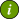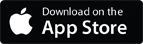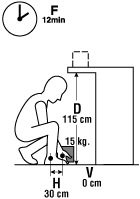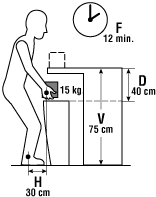### OSH Answers Fact SheetsEasy-to-read, question-and-answer fact sheets covering a wide range of workplace health and safety topics, from hazards to diseases to ergonomics to workplace promotion. MORE ABOUT >Search all fact sheets:

Type a word, a phrase, or ask a question

# Vertical Distance Multiplier Factor

## How can the vertical distance affect the weight limit?

Example: A worker lifts a 13 kg load from loosely piled pieces of metal from the floor to the table five times an hour.To calculate the recommended weight limit (RWL) for the task:

• Determine the weight of the load.

Weight of the object equals 13 kg

• Assess the six components of the lifting task.

H (Horizontal Distance) - 30 cm
V (Vertical Distance) - 0 cm
D (Lifting/Carrying Distance) - 115 cm
F (Frequency) - 12 min
A (Angle) - 0°
C (Coupling/quality of grip) - poor

The revised NIOSH Lifting Equation is: 23 kg x HM x VM x DM x AM x FM x CM = RWL

23 Kg x 0.83 x 0.78 x 0.85 x 1.00 x 1.00 x 0.90 = 11.39 kg

* In this example, the distance was 115 cm. From the tables, the distance factor (D) was greater than 100cm (value of 0.87), so the value of 0.85 was selected (lifting distance of 145 cm) to be more protective. NIOSH does allow for some extrapolation for values that do not match the figures presented in their tables.

• Compare weight of the load against the determined Recommended Weight Limit for the task:

Conclusion

The weight of the load is 13 kg, which is higher than the calculated recommended weight limit of 11.39 kg.

Therefore, this task is likely to increase the risk of a lifting related injury.

### Recommendations

The components: V - starting height, D - lifting distance and H - horizontal distance and C - poor grasp contribute similarly to the risk.

• Raise the metal collection area (starting height) off the floor. This change also decreases D, the lifting distance.The revised NIOSH Lifting Equation is: 23 kg x HM x VM x DM x AM x FM x CM = RWL

23 kg x 0.83 x 0.93* x 0.93 x 1.00 x 1.00 x 0.90 = 14.86 kg

* In this example, the vertical distance is 75 cm. From the tables, the vertical multiplier (VN) was greater than 70 cm (value of 0.99), so the value of 0.93 was selected (lifting distance of 70 cm) to be more protective.

• Compare weight of the load with these adjustments against the Recommended Weight Limit.

The weight of the load at 15 kg is similar to the recommended weight limit of 14.86 kg. Further improvement can be achieved by providing the working materials in containers with handles, or by reducing the weight of the lift.

Therefore, while most workers can likely safely perform the task, the work should be monitored for the development of injuries.

Document last updated on April 27, 2021